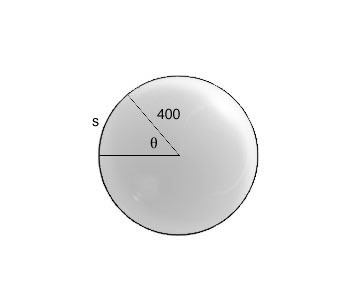SEARCH HOMEMath Central Quandaries & QueriesSamua, a student: Assuming that the Earth is a sphere of radius 4000 miles and that the cities are on the same longitude (one city is due north to the other). Find the distance between the cities with the latitudes of 37 degrees 47'36'' and another city with 47 degrees 37'18''. Heeeeeeeeeeelp!Hi Samua,

The distance from one city to another is an arc length since Earth is approximately a sphere. Derived from the circumference formula and the fact that a circle has 360o, we get the following arc length formula for degrees:

s = (θπr)/180 where θ is the angle between the cities, r is the radius of the Earth and s is the distance between the cities. To find the angle between the cities you will have to subtract their latitudes since both is a measure from the equator and not form each other.Hope this helps,

JaniceMath Central is supported by the University of Regina and The Pacific Institute for the Mathematical Sciences.